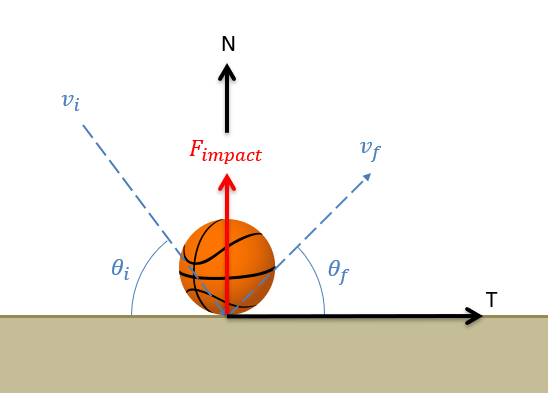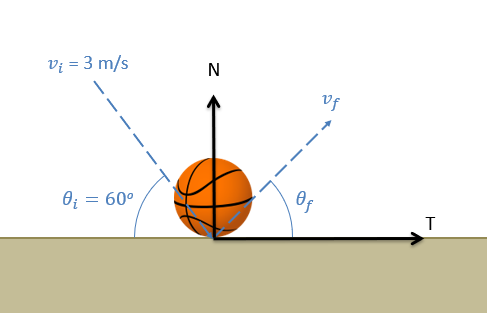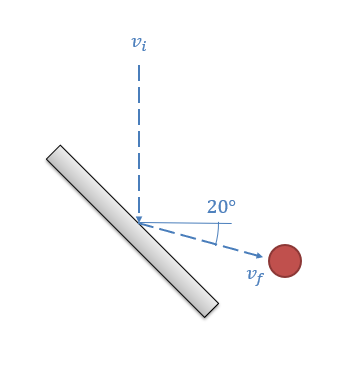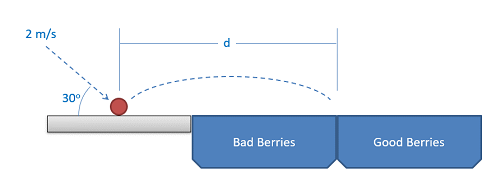﻿ Mechanics Map - Surface Collisions and the Coefficient of Restitution

# Surface Collisions and the Coefficient of Restitution

Here we will use the term surface collision to describe any instance where a body impacts and rebounds off a solid and unmoving surface. A clear example of a surface collision is a basketball bouncing off a hard floor. The basketball will have some velocity before the collision and some second velocity after the collision, with the floor exerting an impulsive force during the collision that causes this change in velocity.

To analyze this collision, we will first need to set up a normal direction (perpendicular to the surface) and a tangential direction (parallel to the surface) for our problem, and break our velocities down into components in these directions. Assuming minimal friction during the impact, we will have an impulsive impact force acting entirely in the normal direction. This fact will form the basis for our analysis.In a surface collision, the impulsive collision force will act in the normal direction. Because there is no force in the tangential direction, the velocity in the tangential direction will not change.

Because the impact force acts entirely in the normal direction, there will be no other significant force to change the momentum of the body in the tangential direction. Assuming the mass remains constant for the body, this means that the velocity must remain constant in the tangential direction because of the conservation of momentum.

 $v_{it}=v_{ft}$

To relate the velocities in the normal direction before and after the collision, we will use something called the coefficient of restitution. The coefficient of restitution is a number between 0 and 1 that measures the "bounciness" of the body and the surface in the collision. Specifically, for a single body being bounced perpendicular off of a surface, the coefficient of restitution is defined as the speed of the body immediately after bouncing off a surface divided by the speed immediately before bouncing off the surface. If we use velocities in place of speed, we will put a negative sign in our equation because the bounce causes a change in direction for the body.

 $e=-\frac{v_{f}}{v_{i}}$

In instances where the body is being bounced off the surface at an angle, the impact force is entirely in the normal direction and the coefficient of restitution relationship specifically applies to the components of the velocities in the normal direction.

 $e=-\frac{v_{nf}}{v_{ni}}$

This relationship can be applied to elastic collisions (where e would be equal to 1), semi-elastic collisions (where e would be some number between 0 and 1) and in-elastic collisions (where e would be equal to 0).

## Worked Problems:

### Question 1:

A tennis ball is dropped from rest from a height of 3 meters. It impacts the ground and bounces back to a maximum height of 2 meters after the impact. What is the coefficient of restitution for the tennis ball on this surface?### Question 2:

A basketball with an initial speed of 3 meters per second impacts a hard floor at the sixty degree angle as shown below. If the collision has a coefficient of restitution of .8, what is the expected speed and angle of the basketball after the impact?### Question 3:

A bounce test is used to sort ripe cranberries from unripe cranberries. In this test cranberries are dropped vertically onto a steel plate sitting at a 45 degree angle. After the impact, a cranberry is observed to bounce off at an angle of 20 degrees below horizontal. Based on this information, what is the coefficient of restitution for the cranberry?### Question 4:

In an alternate cranberry sorter machine, cranberries are launched at a horizontal metal plate with an initial velocity of 2 m/s downwards at a 30-degree angle as shown below. The cranberries then bounce off the plate into one of two baskets, a closer one for bad cranberries and a farther one for good cranberries. If the cranberries are supposed to have a coefficient of restitution of 0.8 or greater, how far away should the edge of the good cranberry bucket be?